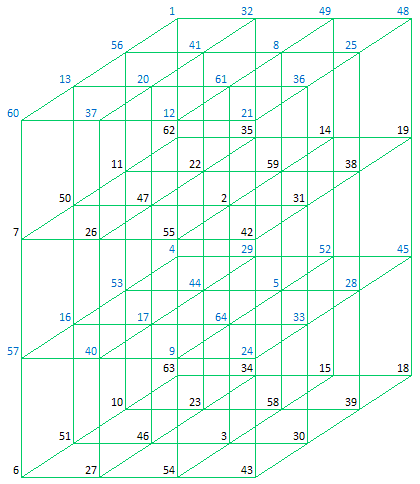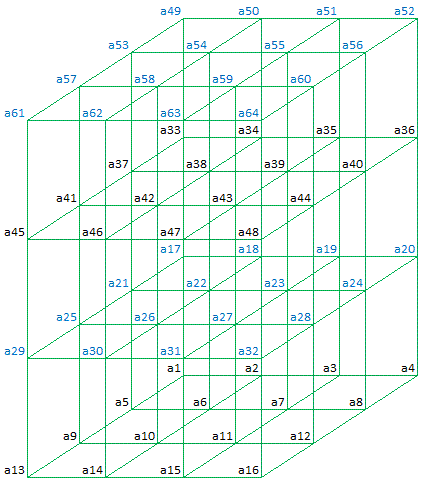Office Applications and Entertainment, Magic CubesIndex About the Author

 3.7   Pantriagonal Magic Cubes (4 x 4 x 4) 3.7.1 Historical Background Magic Cubes as discussed in Section 2.1 for which basically only the rows, columns, pillars and 4 space diagonals (main triagonals) sum to the Magic Constant are normally referred to as Simple Magic Cubes. John Hendricks introduced the concept of Pantriagonal Magic Cubes, being Simple Magic Cubes for which all main and broken triagonals sum to the Magic Constant. In 1972 he published a 4th order Pantriagonal Magic Cube, which is shown below together with the 12 orthogonal planes:Plane 11 (Top)

 1 32 49 48 56 41 8 25 13 20 61 36 60 37 12 21

Plane 21 (Left)

 60 13 56 1 7 50 11 62 57 16 53 4 6 51 10 63

Plane 31 (Back)

 1 32 49 48 62 35 14 19 4 29 52 45 63 34 15 18

Plane 12

 62 35 14 19 11 22 59 38 50 47 2 31 7 26 55 42

Plane 22

 37 20 41 32 26 47 22 35 40 17 44 29 27 46 23 34

Plane 32

 56 41 8 25 11 22 59 38 53 44 5 28 10 23 58 39

Plane 13

 4 29 52 45 53 44 5 28 16 17 64 33 57 40 9 24

Plane 23

 12 61 8 49 55 2 59 14 9 64 5 52 54 3 58 15

Plane 33

 13 20 61 36 50 47 2 31 16 17 64 33 51 46 3 30

Plane 14

 63 34 15 18 10 23 58 39 51 46 3 30 6 27 54 43

Plane 24

 21 36 25 48 42 31 38 19 24 33 28 45 43 30 39 18

Plane 34

 60 37 12 21 7 26 55 42 57 40 9 24 6 27 54 43
 The cube shown above has following additional properties: Compact : Every 2 x 2 sub square in each of the orthogonal planes sums to the Magic Constant. Complete: Every (pan)triagonal contains n/2 complementary pairs spaced n/2 apart along the (pan)triagonal.           Comparable with Most Perfect Magic Squares as discussed in 'Magic Squares', Section 2.4). As a consequence of the defining properties mentioned above, the cube has also following properties: Every 2 x 2 x 2 block of cells (including wrap-around) sums to n*(n3 + 1) = 260 (Cubic Compact). The corners of all Sub-Cubes of orders 3 sum to n*(n3 + 1) = 260. A Pantriagonal Magic Cube can be transformed into another Pantriagonal Magic Cube by moving an orthogonal plane from one side of the cube to the other. (Comparable with the row and column movements for Pandiagonal Magic Squares as discussed in 'Magic Squares' Section 3.4). Consequently a Pantriagonal Magic Cube belongs to a collection {Aijkm} of 43 * 48 = 3072 elements which can be found by means of rotation, reflection or plane movements. The Class of 48 elements which can be obtained by rotation / reflection of a Pantriagonal Cube is shown in Attachment 3.7.1. The Class of 64 elements which can be obtained by planar shifts of a Pantriagonal Cube is shown in Attachment 3.7.2. Each cube of Attachment 3.7.1 can be used as a Base for Attachment 3.7.2. It should be noted that the planar shifts are from left to right (L1, L2, L3), from back to front (B1, B2, B3) and from bottom to top (T1, T2, T3). 3.7.2 Analytic Solution In general Magic Cubes of order 4 can be represented as follows:Plane 11 (Top)

 a49 a50 a51 a52 a53 a54 a55 a56 a57 a58 a59 a60 a61 a62 a63 a64

Plane 21 (Left)

 a61 a57 a53 a49 a45 a41 a37 a33 a29 a25 a21 a17 a13 a9 a5 a1

Plane 31 (Back)

 a49 a50 a51 a52 a33 a34 a35 a36 a17 a18 a19 a20 a1 a2 a3 a4

Plane 12

 a33 a34 a35 a36 a37 a38 a39 a40 a41 a42 a43 a44 a45 a46 a47 a48

Plane 22

 a62 a58 a54 a50 a46 a42 a38 a34 a30 a26 a22 a18 a14 a10 a6 a2

Plane 32

 a53 a54 a55 a56 a37 a38 a39 a40 a21 a22 a23 a24 a5 a6 a7 a8

Plane 13

 a17 a18 a19 a20 a21 a22 a23 a24 a25 a26 a27 a28 a29 a30 a31 a32

Plane 23

 a63 a59 a55 a51 a47 a43 a39 a35 a31 a27 a23 a19 a15 a11 a7 a3

Plane 33

 a57 a58 a59 a60 a41 a42 a43 a44 a25 a26 a27 a28 a9 a10 a11 a12

Plane 14

 a1 a2 a3 a4 a5 a6 a7 a8 a9 a10 a11 a12 a13 a14 a15 a16

Plane 24

 a64 a60 a56 a52 a48 a44 a40 a36 a32 a28 a24 a20 a16 a12 a8 a4

Plane 34

 a61 a62 a63 a64 a45 a46 a47 a48 a29 a30 a31 a32 a13 a14 a15 a16

As all 2 x 2 squares in each of the orthogonal planes sum to 130 and all complementary pairs of all (pan)triagonals sum to 65, this results in linear equations like:

 Plane 11 a49 + a50 + a53 + a54 = 130 a53 + a54 + a57 + a58 = 130 a57 + a58 + a61 + a62 = 130 a61 + a62 + a49 + a50 = 130 a50 + a51 + a54 + a55 = 130 a54 + a55 + a58 + a59 = 130 a58 + a59 + a62 + a63 = 130 a62 + a63 + a50 + a51 = 130 a51 + a52 + a55 + a56 = 130 a55 + a56 + a59 + a60 = 130 a59 + a60 + a63 + a64 = 130 a63 + a64 + a51 + a52 = 130 a52 + a49 + a56 + a53 = 130 a56 + a53 + a60 + a57 = 130 a60 + a57 + a64 + a61 = 130 a64 + a61 + a52 + a49 = 130 Pantriagonal pairs from bottom/left/front to top/right/back a1  + a43 = 65 a5  + a47 = 65 a9  + a35 = 65 a13 + a39 = 65 a30 + a56 = 65 a18 + a60 = 65 a22 + a64 = 65 a26 + a52 = 65 a2  + a44 = 65 a6  + a48 = 65 a10 + a36 = 65 a14 + a40 = 65 a31 + a53 = 65 a19 + a57 = 65 a23 + a61 = 65 a27 + a49 = 65 a3  + a41 = 65 a7  + a45 = 65 a11 + a33 = 65 a15 + a37 = 65 a32 + a54 = 65 a20 + a58 = 65 a24 + a62 = 65 a28 + a50 = 65 a4  + a42 = 65 a8  + a46 = 65 a12 + a34 = 65 a16 + a38 = 65 a29 + a55 = 65 a17 + a59 = 65 a21 + a63 = 65 a25 + a51 = 65

The equations for the other orthogonal planes and pantriagonals are comparable.

The complete set of equations results, after deduction, in following set of linear equations describing the "Pantriagonal Magic Cubes" as published by John Hendricks:

```a(61) =  130 - a(62) - a(63) - a(64)
a(59) =  130 - a(60) - a(63) - a(64)
a(58) =        a(60) - a(62) + a(64)
a(57) =      - a(60) + a(62) + a(63)
a(55) =      - a(56) + a(63) + a(64)
a(54) =        a(56) + a(62) - a(64)
a(53) =  130 - a(56) - a(62) - a(63)
a(52) =  130 - a(56) - a(60) - a(64)
a(51) =        a(56) + a(60) - a(63)
a(50) =  130 - a(56) - a(60) - a(62)
a(49) = -130 + a(56) + a(60) + a(62) + a(63) + a(64)
a(47) =  130 - a(48) - a(63) - a(64)
a(46) =        a(48) - a(62) + a(64)
a(45) =      - a(48) + a(62) + a(63)
a(44) =  130 - a(48) - a(60) - a(64)
a(43) = -130 + a(48) + a(60) + a(63) + 2 * a(64)
a(42) =  130 - a(48) - a(60) + a(62) - 2 * a(64)
a(41) =        a(48) + a(60) - a(62) - a(63) + a(64)
a(40) =        a(48) - a(56) + a(64)
a(39) =  130 - a(48) + a(56) - a(63) - 2 * a(64)
a(38) =        a(48) - a(56) - a(62) + 2 * a(64)
a(37) =      - a(48) + a(56) + a(62) + a(63) - a(64)
a(36) =      - a(48) + a(56) + a(60)
a(35) =        a(48) - a(56) - a(60) + a(63) + a(64)
a(34) =      - a(48) + a(56) + a(60) + a(62) - a(64)
a(33) =  130 + a(48) - a(56) - a(60) - a(62) - a(63)
a(32) =   65 - a(56) - a(62) + a(64)
a(31) = - 65 + a(56) + a(62) + a(63)
a(30) =   65 - a(56)
a(29) =   65 + a(56) - a(63) - a(64)
a(28) = - 65 + a(56) + a(60) + a(62)
a(27) =  195 - a(56) - a(60) - a(62) - a(63) - a(64)
a(26) = - 65 + a(56) + a(60) + a(64)
a(25) =   65 - a(56) - a(60) + a(63)
a(24) =   65 - a(62)
a(23) = - 65 + a(62) + a(63) + a(64)
a(22) =   65 - a(64)
a(21) =   65 - a(63)
a(20) =   65 - a(60) + a(62) - a(64)
a(19) =   65 + a(60) - a(62) - a(63)
a(18) =   65 - a(60)
a(17) = - 65 + a(60) + a(63) + a(64)
a(16) =   65 - a(48) + a(56) + a(62) - 2 * a(64)
a(15) =   65 + a(48) - a(56) - a(62) - a(63) + a(64)
a(14) =   65 - a(48) + a(56) - a(64)
a(13) = - 65 + a(48) - a(56) + a(63) + 2 * a(64)
a(12) =   65 + a(48) - a(56) - a(60) - a(62) + a(64)
a(11) = - 65 - a(48) + a(56) + a(60) + a(62) + a(63)
a(10) =   65 + a(48) - a(56) - a(60)
a( 9) =   65 - a(48) + a(56) + a(60) - a(63) - a(64)
a( 8) =   65 - a(48) + a(62) - a(64)
a( 7) =   65 + a(48) - a(62) - a(63)
a( 6) =   65 - a(48)
a( 5) = - 65 + a(48) + a(63) + a(64)
a( 4) = - 65 + a(48) + a(60) - a(62) + 2 * a(64)
a( 3) =   65 - a(48) - a(60) + a(62) + a(63) - a(64)
a( 2) = - 65 + a(48) + a(60) + a(64)
a( 1) =  195 - a(48) - a(60) - a(63) - 2 * a(64)
```

The linear equations shown above, are ready to be solved, for the Magic Constant 130.

The solutions can be obtained by guessing:

a(48), a(56), a(60), a(62) ... a(64)

and filling out these guesses in the abovementioned equations.

For distinct integers also following relations are applicable:

0 < a(i) =< 64        for i = 1, 2 ... 47, 49 ... 55, 57 ... 59, 61
a(i) ≠ a(j)           for i ≠ j

which have been incorporated in an optimized guessing routine (MgcCube4d).

Subject guessing routine produced 64 * 6 * 120 = 46080 Pantriagonal Magic Cubes within 45 minutes, of which the first 120 are shown in Attachment 3.7.3.

An alternative method to generate Pantriagonal Magic Cubes, based on the equations deducted above, will be discussed in Section 3.12.4.